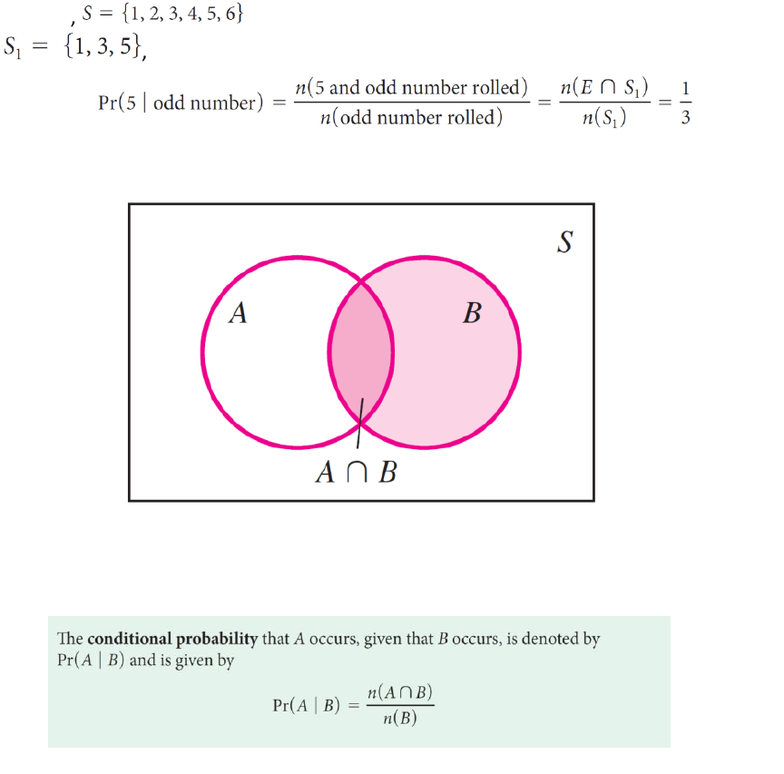# ITM 107 Lecture Notes - Lecture 13: Sample Space, Conditional Probability, Product Rule

317 views3 pagesKATIE CHOI
12/3/18
LECTURE 10 INTRODUCTION TO PROBABILITY
Conditional Probability
Each probability that we have computed has been relative to the sample space for the
experiment.
Sometimes information is given that reduces the sample space needed to solve a stated
problem.
For example, if a die is rolled, the probability that a 5 occurs, given that an odd number
is rolled, is denoted by
Pr(5 rolled | odd number rolled)
The knowledge that an odd number occurs reduces the sample space from the numbers
on a die, , to the sample space for odd numbers on a die,
, so the event E = a 5 occurs” has probability
To find Pr(A | B), we seek the probability that A occurs, given that B occurs.
Figure 7.4 shows the original sample space S with the reduced sample space B shaded.
(Guergachi, 2018)
Because all elements must be contained in B, we evaluate Pr(A | B) by dividing the
number of elements in A B by the number of elements in B.
Condition Probability
(Guergachi, 2018)
Using the definition of Pr(A | B) and dividing the numerator and denominator by n(S),
the number of elements in the sample space, gives
Unlock document

This preview shows page 1 of the document.
Unlock all 3 pages and 3 million more documents.

# Get access

\$10 USD/m
Billed \$120 USD annually
Homework Help
Class Notes
Textbook Notes